# JP5582920B2 - Image processing device - Google Patents

## Info

Publication number
JP5582920B2
JP5582920B2 JP2010186023A JP2010186023A JP5582920B2 JP 5582920 B2 JP5582920 B2 JP 5582920B2 JP 2010186023 A JP2010186023 A JP 2010186023A JP 2010186023 A JP2010186023 A JP 2010186023A JP 5582920 B2 JP5582920 B2 JP 5582920B2
Authority
JP
Japan
Prior art keywords
value
image processing
processing apparatus
resolution
pixel
Prior art date
Legal status (The legal status is an assumption and is not a legal conclusion. Google has not performed a legal analysis and makes no representation as to the accuracy of the status listed.)
Active
Application number
JP2010186023A
Other languages
Japanese (ja)
Other versions
JP2012043336A (en
JP2012043336A5 (en
Inventor

Original Assignee

Priority date (The priority date is an assumption and is not a legal conclusion. Google has not performed a legal analysis and makes no representation as to the accuracy of the date listed.)
Filing date
Publication date
Application filed by 三菱電機株式会社 filed Critical 三菱電機株式会社
Priority to JP2010186023A priority Critical patent/JP5582920B2/en
Publication of JP2012043336A publication Critical patent/JP2012043336A/en
Publication of JP2012043336A5 publication Critical patent/JP2012043336A5/ja
Application granted granted Critical
Publication of JP5582920B2 publication Critical patent/JP5582920B2/en
Application status is Active legal-status Critical
Anticipated expiration legal-status Critical

## Images

•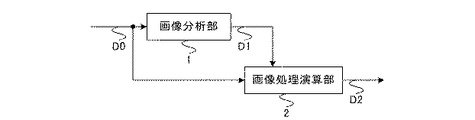•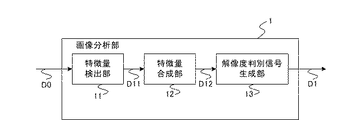•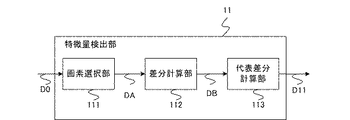•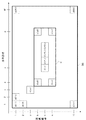•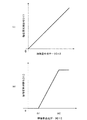•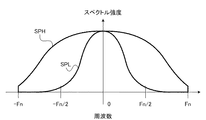•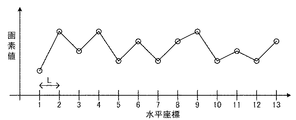•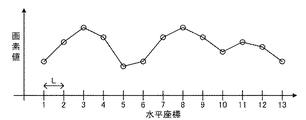•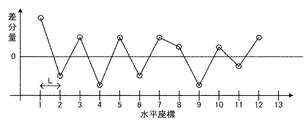•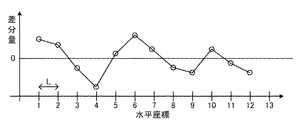•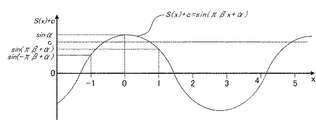•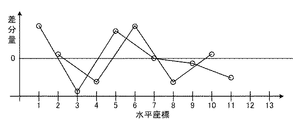•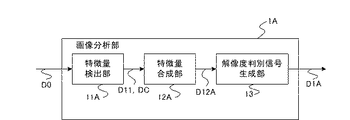•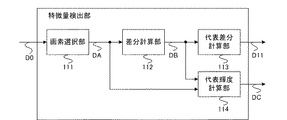•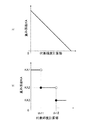•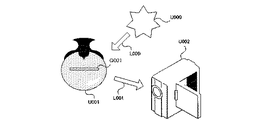•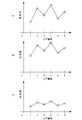•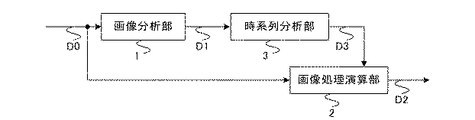•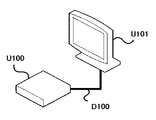•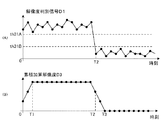•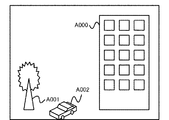•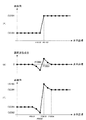•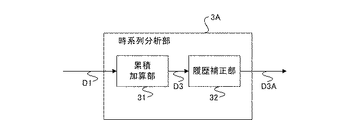•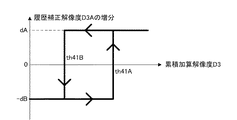•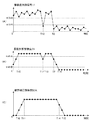## Classifications

• GPHYSICS
• G06COMPUTING; CALCULATING; COUNTING
• G06KRECOGNITION OF DATA; PRESENTATION OF DATA; RECORD CARRIERS; HANDLING RECORD CARRIERS
• G06K9/00Methods or arrangements for reading or recognising printed or written characters or for recognising patterns, e.g. fingerprints
• GPHYSICS
• G06COMPUTING; CALCULATING; COUNTING
• G06TIMAGE DATA PROCESSING OR GENERATION, IN GENERAL
• G06T5/00Image enhancement or restoration
• G06T5/001Image restoration
• G06T5/002Denoising; Smoothing
• GPHYSICS
• G06COMPUTING; CALCULATING; COUNTING
• G06TIMAGE DATA PROCESSING OR GENERATION, IN GENERAL
• G06T5/00Image enhancement or restoration
• G06T5/20Image enhancement or restoration by the use of local operators
• GPHYSICS
• G06COMPUTING; CALCULATING; COUNTING
• G06TIMAGE DATA PROCESSING OR GENERATION, IN GENERAL
• G06T2207/00Indexing scheme for image analysis or image enhancement
• G06T2207/10Image acquisition modality
• G06T2207/10016Video; Image sequence

## Description

The present invention relates to an image processing apparatus.

An image correction is automatically performed by detecting the resolution of the input image. For example, in the automatic correction circuit according to Patent Document 1, resolution detection means for detecting the resolution of an image, setting value calculation means for calculating a setting value used for image correction based on the detected resolution, and based on the calculated setting value And image correction means for performing image correction. In order to detect the resolution of the image, the period from the rising edge to the falling edge of the enable signal indicating the effective period of the image is counted.

JP 2006-166188 A (FIG. 1, FIG. 2, FIG. 3)

Counting the period from the rising edge to the falling edge of the enable signal representing the effective period of the image is equivalent to counting the number of horizontal pixels or vertical pixels of the image. That is, the technique of Patent Document 1 detects the resolution of the input image from the number of pixels of the input image.
However, if the resolution of the image is detected from the number of pixels of the image as described above, the resolution may not be detected correctly depending on the image. That is, when an image obtained by enlarging a certain low-resolution image is input as an input image, it is appropriate to consider that the resolution of the input image is lower than the resolution that can be considered from the number of pixels. Therefore, if image correction is performed based on the number of pixels of the input image, it cannot be appropriately processed depending on the input image.

The image processing apparatus according to the present invention determines how much a signal component having a predetermined frequency or more determined from a predetermined interval is included in the input image based on a change in pixel values of a plurality of pixels located at a predetermined interval in the input image. An image processing apparatus including an image analysis unit that obtains a resolution determination signal to be displayed, wherein the image analysis unit includes a plurality of pixels that are located at predetermined intervals with respect to each pixel existing in a predetermined region of the input image. A feature amount detection unit that obtains and outputs a representative difference value from the pixel value of each pixel, and a feature amount synthesis unit that obtains and outputs a feature amount composite value from the representative difference value obtained for each pixel existing in a predetermined region; A resolution discriminating signal generating unit that obtains and outputs a resolution discriminating signal having a monotonous non-decreasing relationship with the feature amount synthesized value from the feature amount synthesized value, and the feature amount detecting unit is located within a predetermined region of the input image Pre-existing picture Each pixel is set as a pixel of interest, N pixels arranged at a predetermined interval in a predetermined direction with the pixel of interest as a reference are set as a pixel of interest, and given by the N pixels constituting the pixel of interest A pixel selection unit that outputs N pixel values as target pixel group pixel value data, and N−1 that is a difference between two adjacent pixel values from N pixel values that constitute the target pixel group pixel value data. A difference calculation unit that calculates the number of difference amounts, and when the code of the N−1 difference amounts is a predetermined pattern, outputs the minimum value of the absolute values of the N−1 difference amounts as a representative difference value, In other cases, a representative difference calculation unit that outputs zero as a representative difference value is provided.
According to another image processing apparatus of the present invention, a signal component having a frequency equal to or higher than a predetermined frequency determined from a predetermined interval based on a change in pixel values of a plurality of pixels located at a predetermined interval in an input image with a moving image as an input. An image processing apparatus including an image analysis unit that obtains a resolution determination signal indicating how much is included in an input image, wherein the image analysis unit uses the pixel as a reference for each pixel existing in a predetermined region of the input image A feature amount detection unit that obtains and outputs a representative difference value from pixel values of a plurality of pixels located at a predetermined interval, and obtains a feature amount composite value from the representative difference value obtained for each pixel existing in the predetermined region. For each image constituting a moving image, and a resolution discriminating signal generating unit for obtaining and outputting a resolution discriminating signal having a monotonic non-decreasing relationship with respect to the feature amount synthesizing value from the feature amount synthesizing value Image analysis department Resolution discrimination signal obtained is input in time series, it is characterized in that comprises a sequence analyzer when generating the time series processed resolution discrimination signal.

According to the image processing apparatus of the present invention, an input image can be processed according to the resolution of the input image.

1 is a configuration diagram of an image processing apparatus according to Embodiment 1 of the present invention. It is a block diagram of the image analysis part which the image processing apparatus which concerns on Embodiment 1 of this invention has. It is a block diagram of the feature-value detection part which the image processing apparatus which concerns on Embodiment 1 of this invention has. It is a figure explaining the pixel in the input image input into the image processing apparatus which concerns on this invention. It is a figure explaining the operation | movement of the resolution discrimination | determination signal generation part which the image processing apparatus which concerns on Embodiment 1 of this invention has. It is a figure explaining the frequency spectrum of the input image input into an image processing device. It is a figure showing the change of the pixel value of the input image input into the image processing apparatus which concerns on Embodiment 1 of this invention. It is a figure showing another change of the pixel value of the input image input into the image processing apparatus which concerns on Embodiment 1 of this invention. It is a figure which shows the difference of the pixel value of the input image of FIG. 7 input into the image processing apparatus which concerns on Embodiment 1 of this invention. It is a figure which shows the difference of the pixel value of the input image of FIG. 8 input into the image processing apparatus which concerns on Embodiment 1 of this invention. It is a figure explaining the influence of the low frequency component to the code | symbol of the difference of a pixel value becoming alternate in the image processing apparatus which concerns on Embodiment 1 of this invention. It is a figure which shows the difference with the pixel used as 2 pixels of the pixel value of the input image of FIG. 8 input into the image processing apparatus which concerns on Embodiment 1 of this invention. It is a block diagram of the image analysis part which the image processing apparatus which concerns on Embodiment 2 of this invention has. It is a block diagram of the feature-value detection part which the image processing apparatus which concerns on Embodiment 2 of this invention has. It is a figure explaining how to obtain | require a weighting coefficient with the image processing apparatus which concerns on Embodiment 2 of this invention. It is a figure showing an example of the environment for acquiring an input image with the image processing apparatus which concerns on Embodiment 2 of this invention. It is a figure which considers the input image imaged with different brightness with the image processing apparatus which concerns on Embodiment 2 of this invention. It is a block diagram of the image processing apparatus which concerns on Embodiment 3 of this invention. It is a figure explaining the utilization method of the image processing apparatus which concerns on Embodiment 3 of this invention by an example. It is a figure explaining operation | movement of the time series analysis part which the image processing apparatus which concerns on Embodiment 3 of this invention has. It is a figure of the input image for demonstrating operation | movement of the image processing apparatus which concerns on Embodiment 3 of this invention by an example. It is a figure explaining the overshoot and undershoot which generate | occur | produce in the emphasis process in an image processing device. It is a block diagram of the time series analysis part which the image processing apparatus which concerns on Embodiment 4 of this invention has. It is a figure explaining operation | movement of the log | history correction part which the image processing apparatus which concerns on Embodiment 4 of this invention has. It is a figure explaining operation | movement of the log | history correction part which the image processing apparatus which concerns on Embodiment 4 of this invention has.

Embodiment 1 FIG.
FIG. 1 is a configuration diagram of an image processing apparatus according to Embodiment 1 of the present invention. The image processing apparatus according to Embodiment 1 includes an image analysis unit 1 that obtains the resolution of an input image D0, and an image processing calculation unit 2 that processes the input image D0 according to the obtained resolution to obtain an output image D2.

The image analysis unit 1 obtains the resolution of the input image D0 from the pixel value of the input image D0, and outputs the result as a resolution discrimination signal D1. The image processing calculation unit 2 performs various image processing such as noise processing and enhancement processing on the input image D0 using the resolution determination signal D1, and outputs the resulting image as the output image D2. Here, the image processing calculation unit 2 changes the image processing operation according to the value of the resolution determination signal D1.
Although details will be described later, the image analysis unit 1 receives a signal component having a frequency equal to or higher than a predetermined frequency determined from the predetermined interval based on a change in pixel values of a plurality of pixels located at the predetermined interval in the input image D0. The resolution indicating how much is included in the image is obtained.

FIG. 2 is a diagram illustrating the configuration of the image analysis unit 1. The image analysis unit 1 includes a feature amount detection unit 11, a feature amount synthesis unit 12, and a resolution determination signal generation unit 13.

First, the feature amount detection unit 11 will be described. For each pixel existing in a predetermined area of the input image D0, the feature amount detection unit 11 sets and sets a target pixel group that is a set of a plurality of pixels arranged at a predetermined interval with reference to the pixel. A representative difference value D11 is obtained and output by a process described later from the change in the pixel values of the pixels constituting the pixel group of interest.

FIG. 3 is a diagram illustrating the configuration of the feature amount detection unit 11. The feature amount detection unit 11 includes a pixel selection unit 111, a difference calculation unit 112, and a representative difference calculation unit 113.

The pixel selection unit 111 outputs, for each pixel existing in a predetermined area of the input image D0, the pixel value of the pixel constituting the target pixel group set for each pixel as the target pixel group pixel value data DA.

FIG. 4 is a diagram for explaining pixels in an input image input to the image processing apparatus according to the present invention. The operation of the pixel selection unit 111 and the target pixel group pixel value data DA will be described with reference to FIG. FIG. 4 schematically shows the input image D0 and the pixels constituting the input image D0. The smallest square in FIG. 4 represents a pixel. Further, horizontal coordinates and vertical coordinates are defined along the horizontal direction and the vertical direction of the input image D0, and the pixel value of the pixel located at the coordinate represented by x and y is represented by d (x , Y). Further, the interval between the lattice points of these coordinate axes is assumed to be equal to the interval between the pixels constituting the input image D0. In the example shown in FIG. 4, the number of pixels in the horizontal direction of the input image D0 is W, and the number of pixels in the vertical direction is H.

Picture element selection unit 111, as expressed by the horizontal coordinate x in FIG. 4, the pixels represented by the vertical coordinate y (x, y), ( a, b), (p, b), (a, q) , The target pixel group and the target pixel group pixel value data DA are obtained for each pixel existing in a region R represented by a rectangle having four pixels represented by (p, q) as vertices. Here, the target pixel group has four pixels arranged in the horizontal direction at intervals of one pixel with respect to each pixel existing in the region R as a starting point. That is, the target pixel group for the target pixel represented by (u, v) has four pixels (u, v), (u + 1, v), (u + 2, v), and (u + 3, v). Thus, the target pixel group pixel value data DA has four pixel values d (u, v), d (u + 1, v), d (u + 2, v), and d (u + 3, v).

If the region R is set so that at least three pixels remain at the right end of the screen, the case-by-case processing such as whether or not there is a pixel in (u + 3, v) becomes unnecessary, and the processing becomes simple. The region R is preferably set so as to include the center of the screen that the viewer often sees. If the area R is reduced, the resolution determination process can be accelerated, and if the area R is increased, the resolution of the image can be determined more accurately than when the area R is small.

The operation of the difference calculation unit 112 will be described. The difference calculation unit 112 calculates one difference amount (pixel value change amount) for each pair of two adjacent pixels among the four pixels. That is, the difference amounts S (1), S (2), S (3) calculated by the following formula are calculated, and the result is output as the difference data DB.
S (1) = d (u + 1, v) −d (u, v)
S (2) = d (u + 2, v) −d (u + 1, v)
S (3) = d (u + 3, v) −d (u + 2, v)

When the difference data DB is arranged in the order of S (1), S (2), and S (3), the representative difference calculation unit 113 has a value other than zero, for example, when a positive value and a negative value are alternately arranged. The smallest value among the absolute values of S (1), S (2), and S (3) is output as the representative difference value D11. In other cases, zero is output as the value of the representative difference value D11.

More specifically, when S (1) is a positive value and S (2) is a negative value and S (3) is a positive value, or S (1) is a negative value and S (2) When S is a positive value and S (3) is a negative value, the smallest value of the absolute values of S (1), S (2), and S (3) is output as the representative difference value D11. In this case, zero is output as the value of the representative difference value D11.

The feature amount calculation unit 11 outputs the representative difference value D11 obtained by the above procedure for each pixel included in the region R. In the example of FIG. 4, since (p−a + 1) × (q−b + 1) pixels are included in the region R, (p−a + 1) × (q−b + 1) representative difference values D11 are output. .

Next, the operation of the feature amount combining unit 12 will be described. The feature quantity synthesis unit 12 outputs a feature quantity synthesis value obtained by synthesizing the representative difference value D11 output from the feature quantity calculation unit 11 as feature quantity synthesis data D12. Here, the feature quantity synthesis unit 12 outputs a value obtained by adding (p−a + 1) × (q−b + 1) representative difference values D11 as the feature quantity synthesis data D12.

Next, the operation of the resolution determination signal generator 13 will be described. The resolution determination signal generation unit 13 outputs the resolution determination signal D1 that is monotonously non-decreasing with respect to the value of the feature amount composite data D12.

Monotonic non-decrease means: Considering two values D12X and D12Y as the value of the feature amount composite data D12, the value of the resolution discrimination signal D1 output when the value of the feature amount composite data D12 is D12X is D1X, and the value of the feature amount composite data D12 is When the value of the resolution discrimination signal D1 output in the case of D12Y is represented by D1Y, if D12X <D12Y, D1X ≦ D1Y.

FIG. 5 is a diagram for explaining the operation of the resolution determination signal generator included in the image processing apparatus according to Embodiment 1 of the present invention. FIG. 5A shows a case where the resolution discrimination signal D1 is output by the resolution discrimination signal D1 so as to be proportional to the feature amount synthesis data D12. In FIG. 5B, the resolution discrimination signal D1 is set to zero when the feature amount synthesis data D12 is equal to or less than the threshold value th1, and the resolution discrimination signal D1 is expressed by a linear function from the threshold value th1 to the threshold value th2. In addition, the resolution discrimination signal generation unit 13 operates so that the resolution discrimination signal D1 becomes a constant value when the feature amount synthesis data D12 is equal to or greater than the threshold th2.

Since the feature amount composite data D12 is the sum of a large number of representative difference values D11, it becomes a large value and needs to be expressed with a large number of bits. The resolution determination signal D1 is a predetermined number of bits (for example, 8 bits). By converting the feature amount combined data D12 into the resolution determination signal D1 with a monotonous non-decreasing relationship, it is possible to obtain a resolution determination signal D1 that can be easily processed by the image processing calculation unit 2.

Hereinafter, functions and effects of the image analysis unit 1 and effects of the image processing apparatus according to the first embodiment of the present invention will be described.

FIG. 6 is a diagram illustrating the frequency spectrum of the input image input to the image processing apparatus. In FIG. 6, Fn represents the Nyquist frequency determined from the number of pixels per unit length of the input image D0. When the input image D0 has only a signal having a frequency of Fn, the same pixel value is alternately taken in the horizontal direction and the vertical direction.

Since the spectral intensity of the input image D0 is given for each point on the frequency plane extended by two frequency axes corresponding to the horizontal frequency and the vertical frequency, a three-dimensional space is used to accurately describe the shape. It is necessary to use it. However, since the shape spreads isotropically around the origin of the frequency axis, the shape can be schematically represented by using one frequency axis. Therefore, only one frequency axis is used in FIG. When the pixel interval is different between the horizontal direction and the vertical direction, it is necessary to consider both the horizontal frequency and the vertical frequency.

Generally, the spectral intensity of the input image D0 has a peak at the origin (frequency is zero), and the spectral intensity decreases as the frequency increases. When an image is picked up by the image pickup device, a frequency component larger than the Nyquist frequency determined from the number of image pickup devices per unit length is not included in the image. Therefore, the frequency spectrum of the input image D0 has a certain spectrum intensity up to a band where the frequency takes a value in the vicinity of ± Fn, as represented by the spectrum intensity SPH, and becomes zero when Fn or more.

However, depending on the input image D0, the frequency spectrum may not have the shape indicated by the spectral intensity SPH. As a typical example, it is conceivable that the input image D0 is obtained by enlarging an image having a smaller number of pixels than the input image D0. For example, when an input image D0 is obtained by enlarging an image having the number of pixels of W / 2 pixels in the horizontal direction and H / 2 pixels in the vertical direction by doubling both in the horizontal direction and the vertical direction, the frequency of the input image D0 The spectrum is as indicated by the spectral intensity SPL. That is, the spectrum intensity is maintained at a certain value until the frequency is in the vicinity of ± Fn / 2, but is almost zero in the frequency band where the absolute value of the frequency is sufficiently larger than Fn / 2.

Consider the case where the image processing operation unit 2 performs enhancement processing. A simple enhancement process is realized by extracting a high frequency component near a specific frequency band of the input image D0, amplifying it with a predetermined gain, and adding it to the input image D0. The reason for this processing is that the spectral intensity of the high frequency component of the input image D0 is increased by the above processing. Note that a high frequency component pass filter, a band frequency component pass filter, or the like can be used to extract a frequency component near a specific frequency band.

In the case where the frequency spectrum of the input image D0 is represented by the spectral intensity SPH, frequency components in a predetermined band having an absolute frequency value of about Fn / 2 or more and Fn or less are extracted and amplified with a predetermined gain. Thereafter, the spectral intensity of the high frequency component can be increased by adding to the input image D0.

On the other hand, in the case where the frequency spectrum of the input image D0 is represented by the spectral intensity SPL, the absolute value of the frequency is about Fn / 2 or more, and the corresponding frequency component in the first place is slightly smaller than Fn. Since it is not included, extracting the frequency component in this band has little effect. Instead, for example, it is more effective to extract a frequency component in a predetermined band whose absolute value of frequency is about Fn / 4 or more and Fn / 2 or less, amplify it with a predetermined gain, and add it to the input image D0. is there.

In short, both the input image D0 giving the spectral intensity SPH and the input image D0 giving the spectral intensity SPL have the same number of pixels, but the frequency spectrum spreads differently, so the contents of effective image processing are different for each. . Therefore, it is desirable to change the processing content of the image processing calculation unit 2 according to how the frequency spectrum spreads instead of the number of pixels of the input image D0, for example, to change the frequency characteristics of the high frequency component pass filter.

Instead of changing the frequency characteristics of the high frequency component pass filter, the gain for the extracted frequency component may be controlled. For example, the high frequency component pass filter is designed to extract a high frequency component in a band where the absolute value of the frequency is equal to or greater than a value near Fn / 2, and a frequency spectrum having a shape represented by the spectrum intensity SPH is obtained. The gain value may be decreased for an input image having the gain, and the gain value may be increased for an input image having a frequency spectrum having a shape represented by the spectrum intensity SPL.

In other words, an image corresponding to the resolution of the input image D0 is obtained by obtaining a signal indicating how much the frequency spectrum of the input image D0 is spread or a predetermined frequency band. Processing can be performed.

However, in order to accurately calculate the frequency spectrum of the input image D0, it is necessary to Fourier transform the input image D0, and the amount of calculation becomes enormous. Therefore, in order to realize the process of changing the calculation content of the image processing calculation unit 2 according to the spread of the frequency spectrum of the input image D0, a method of estimating the spread of the frequency spectrum of the input image D0 by a method other than Fourier transform is necessary.

According to the image analysis unit 1 of the first embodiment of the present invention, the spread of the frequency spectrum of the input image D0 can be estimated with a simple calculation. Therefore, in the image processing apparatus according to the first embodiment of the present invention, it is possible to change the calculation content of the image processing calculation unit 2 according to how the frequency spectrum of the input image D0 spreads.

It will be apparent to those skilled in the art from the description of the first embodiment that the amount of calculation of the image analysis unit 1 is smaller than the Fourier transform of the input image D0. Therefore, hereinafter, it will be described that the shape of the frequency spectrum of the input image D0 can be estimated by the image analysis unit 1.

7 and 8 are broken lines representing data obtained by plotting the pixel values of the input image D0 for 13 pixels in the horizontal direction. Here, FIG. 7 shows an example in which the change in the pixel value includes many changes that can be approximated by a sine wave represented by a frequency of about ± Fn, and FIG. 8 shows the change in the pixel value by ± Fn / This is an example in which many changes that can be approximated by a sine wave represented by a frequency of about 2 are included. 7 and 8, the distance between the pixels of the input image D0 is represented by L.

Hereinafter, the feature amount calculation unit 11 sets a pixel group of interest in the procedure described above for pixels whose horizontal coordinates are 1 to 10 among the pixels shown in FIGS. It is assumed that the representative difference value D11 is obtained. FIG. 9 shows a difference amount obtained by subtracting the pixel value of a pixel having a large coordinate from its own pixel value with respect to FIG. FIG. 10 shows the difference amount obtained with respect to FIG.

As can be seen from the difference amount shown in FIG. 9, the signs of the three consecutive difference amounts in the example of the pixel value shown in FIG. 7 are alternately (+ − + or − ++ −), and the representative difference value D11. The pixel group of interest for which takes a value other than zero is as follows.
A pixel group of interest set for a pixel given by horizontal coordinate 1 A pixel group of interest set for a pixel given by horizontal coordinate 2 A pixel group of interest set for a pixel given by horizontal coordinate 3 A pixel group of interest set for a pixel given by 4 A pixel group of interest set for a pixel given by horizontal coordinate 5 A pixel group of interest set for a pixel given by horizontal coordinate 8 A pixel group of interest set for a given pixel A pixel group of interest set for a pixel given by a horizontal coordinate 10

In this case, since the representative difference value D11 often takes a value other than zero, the feature amount composite data D12 obtained by adding the representative difference value D11 takes a value that is somewhat large.

On the other hand, as can be seen from the difference amount shown in FIG. 10, in the example shown in FIG. 8, the representative difference value D11 calculated from the target pixel group set for the pixel shown below takes a value other than zero.
The pixel group of interest set for the pixel given by the horizontal coordinate 9

In this case, there is only one pixel out of 10 pixels when the value of the representative difference value D11 takes a value other than zero, and the feature amount composite data D12 obtained by adding the representative difference value D11 does not increase.
In the above example, when the change in the pixel value of the input image D0 includes many components that can be approximated by a sine wave whose absolute value of the frequency is about Fn, the value of the feature amount composite data D12 becomes large, and the input value It is shown that when the change in the pixel value of the image D0 includes many components that can be approximated by a sine wave whose absolute value of frequency is lower than Fn, for example, about Fn / 2, the value is not so large. However, as a result of the following consideration, the change in the pixel value of the input image D0 includes more components that can be approximated by a sine wave whose absolute value of the frequency is greater than about Fn / 2. It can be seen that the value increases.

When the input image D0 includes a frequency component of ± β * Fn (referred to as a frequency of interest, 0 <β ≦ 1), the difference frequency S also includes a component of the frequency of interest. Assuming that only the component of the target frequency exists in the difference amount S, the phase of the three consecutive difference amounts S is −πβ + α, where α (0 or more and less than π) is the phase of the central difference amount S. α, πβ + α. The conditions under which the signs of the three difference amounts S are alternate are as follows.
−π <−πβ + α <0 <α <π <πβ + α <2π (1)
From the expression (1), the following expression (2) is obtained.
1/2 <β ≦ 1 and π (1-β) <α <πβ (2)

From equation (2), it can be seen that if β is greater than ½, as the β is closer to 1, the sign of the three difference amounts S alternates with a greater probability (approximately 100% when β = 1). When only the component of the frequency of interest exists in the difference amount S, it indicates that the signs of the three consecutive difference amounts S do not occur alternately at the frequency of interest having an absolute value of Fn / 2 or less. Yes. Actually, a frequency other than the frequency of interest is included in the input image D0, and there are cases where the signs of the three consecutive difference amounts S are alternated even with a frequency whose absolute value is not more than Fn / 2. However, the lower the frequency, the smaller the probability of occurrence of the case where the codes of three consecutive difference amounts S alternate.

Consider a case in which since there are signals of a plurality of frequencies, the signs of three consecutive difference amounts S alternate even with only a signal component having a frequency whose absolute value is Fn / 2 or less. Consider a case where there are two signals of the frequency of interest and another frequency (frequency γFn, phase δ).
S (x) = sin (πβ * x + α) + b * sin (πγ * x + δ) (3)
Here, x is a pixel position. Pixels with different x are adjacent to each other. If γ is sufficiently small and hardly changes in one period of the frequency of interest, the second term on the right side of equation (3) is replaced with a variable (−c) as follows.
S (x) + c = sin (πβ * x + α) (4)
Although only one frequency γFn is shown in the expression (3), the influence of low frequency components (all frequencies below about a fraction of β) can be expressed as a variable c. Since the difference amount S is an amount corresponding to the first-order differentiation of the pixel value, the value of the difference amount S becomes smaller at the same amplitude as the frequency is lower. Since the spectrum distribution as shown in FIG. 6 is assumed, as β increases, a larger frequency is included in the low-frequency component, and the variable c can take a larger value.

FIG. 11 is a diagram for explaining the influence of low-frequency components on the alternating signs of pixel value differences in the image processing apparatus according to Embodiment 1 of the present invention. FIG. 11 shows the state of equation (4) near x = 0. In FIG. 11, the sign of the difference amount S (x) at three pixels of x = −1, 0, 1 is − + −. Although the details are omitted, the conditions under which the sign of the difference amount alternates in three pixels are as follows.
π (1-β) / 2 <α ≦ π / 2, and sin (πβ + α) <c <sin (α) (5A)
π / 2 ≦ α <π (1 + β) / 2 and sin (−πβ + α) <c <sin (α) (5B)

From the formulas (5A) and (5B), even if β is a value close to 0, the sign of the difference amount may be alternated by 3 pixels, and as β is larger, the sign of the difference amount is alternated by 3 pixels. The range of α is large, and it can be seen that the range of the variable c is large when α is fixed. Further, as α is closer to π / 2, the range of the variable c in which the sign of the difference amount is alternated with three pixels even when β is the same is increased.
The value of the representative difference value D11 includes the influence of the frequency corresponding to β close to 0, but compared to the influence of the frequency where β is sufficiently larger than 1/2, the influence of the frequency corresponding to β close to 0 The size is much smaller.

From the above, the resolution discriminating signal D1 that takes a non-zero value when the signs of the three consecutive difference amounts S are alternated has an absolute value of about Fn / 2 or more (a frequency smaller than Fn / 2 is also included). It is understood that this means that a value obtained by weighting and adding the frequency of (including) is weighted and added with a weighting factor that increases as the frequency increases. Then, it can be seen that the feature amount synthesis data D12 becomes larger as the change in the pixel value of the input image D0 includes more components that can be approximated by a sine wave having a frequency whose absolute value is about Fn / 2 or more.
Further, since the resolution discrimination signal D1 is monotonously non-decreasing with respect to the feature amount composite data D12, the resolution discrimination signal D1 also has a frequency whose absolute value of the frequency is about Fn / 2 or more due to a change in the pixel value of the input image D0. The larger the component that can be approximated by a sine wave, the larger the value. In addition, when the change in the pixel value of the input image D0 includes many components that can be approximated by a sine wave having a frequency whose absolute value is about Fn / 2 or more, the frequency spectrum of the input image D0 has an absolute value of Fn. It extends to the band where the value is more than / 2. Therefore, it is possible to estimate to what extent the frequency spectrum of the input image D0 is spread by the value of the resolution discrimination signal D1.

Further, since it is possible to estimate to what frequency band the frequency spectrum of the input image D0 has spread based on the value of the resolution determination signal D1, by using the resolution determination signal D1, an image suitable for the frequency spectrum of the input image D0. Processing can be performed.

In the above description, when the change in the pixel value of the input image D0 includes many components that can be approximated by a sine wave having a frequency whose absolute value is about Fn / 2 or more, the resolution determination signal D1 has a large value. However, the frequency band to which the resolution determination signal D1 responds is not limited to this example. By changing the configuration of the pixel group of interest, the frequency band in which the absolute value of the frequency is smaller than Fn / 2 can be made the frequency band to which the resolution determination signal D1 reacts.

Hereinafter, an example will be described in which the frequency spectrum of the input image D0 determines how many frequency components are in a band where the absolute value of the frequency is about Fn / 4 or more and about Fn / 2 or less. In this case, the target pixel group given to each pixel in the region R may be four pixels arranged in the horizontal direction at intervals of two pixels starting from itself.

That is, the target pixel group set for the pixel represented by (u, v) has four (u, v), (u + 2, v), (u + 4, v), and (u + 6, v). Will have pixels.
Further, the target pixel group pixel value data DA has four pixel values d (u, v), d (u + 2, v), d (u + 4, v), and d (u + 6, v).
Further, the difference data DB has three difference amounts S (1), S (2), and S (3) expressed by the following equations.
S (1) = d (u + 2, v) −d (u, v)
S (2) = d (u + 4, v) −d (u + 2, v)
S (3) = d (u + 6, v) −d (u + 4, v)

When the frequency spectrum of the input image D0 has a large number of components in the vicinity of ± Fn / 2 among the frequency components in the band where the absolute value of the frequency is about Fn / 4 or more and about Fn / 2 or less, FIG. As shown in FIG. 5, the change in the pixel value of the input image D0 can be approximated by a sine wave having a period of about 4L. FIG. 12 shows the difference between the pixel value of the right two pixels and the pixel value of FIG.

As can be seen from FIG. 12, when the representative difference value D11 is calculated for the horizontal coordinate from 1 to 7 in FIG. 8, the representative difference value D11 calculated from the pixel group of interest shown below is Takes a non-zero value.
Horizontal coordinate 1
Horizontal coordinate 2
Horizontal coordinate 4
Horizontal coordinate 6

It can be seen that by taking the difference at intervals of 2 pixels, the opportunity for the representative difference value D11 to take a value other than zero is increased compared to the case of taking the difference at intervals of 1 pixel. In accordance with this, it is also clear that the feature amount composite data D12 has a certain value and the value of the resolution determination signal D1 also increases. Therefore, it can be determined from the resolution discrimination signal D1 how many components of the frequency spectrum of the input image D0 can be approximated by a sine wave having a frequency whose absolute value is about Fn / 4 or more and about Fn / 2 or less. I understand.

From the above example, the frequency spectrum of the input image D0 can be obtained by setting the pixel group of interest given to each pixel in the region R to four pixels arranged in the horizontal direction at two pixel intervals starting from itself. It can be seen that it is possible to determine whether or not at least the frequency ranges from about ± Fn / 4 or more to a band in the vicinity of ± Fn / 2.

From the above description, when the image analysis unit 1 determines to which frequency range the frequency spectrum of the input image D0 extends, the interval between the pixels constituting the pixel group of interest is determined by the frequency band to be determined. It can be seen that it may be set appropriately. In particular, the closer the frequency band to be determined is to the lower frequency side, the wider the interval between the pixels constituting the pixel group of interest.

In the above description, the number of pixels constituting the target pixel group is 4, but the number of pixels may be 3 or 5 or more. When the pixel group of interest is composed of three pixels, it becomes easier to obtain a larger resolution discrimination signal D1. However, a component having an absolute value of frequency of Fn / 2 or less also contributes to the resolution determination signal D1 at a higher rate than in the case of 4 pixels. When the pixel group of interest is composed of 5 pixels or more, the contribution of the component whose absolute value of frequency is far from Fn is smaller than that of the case of 4 pixels, and the component whose absolute value of frequency is closer to Fn is resolved with a large weight. This can contribute to the discrimination signal D1. When 5 pixels are used, the frequency whose absolute value is about Fn * (2/3) or more is mainly detected.
Furthermore, although the pixel group of interest is composed of pixels arranged in the horizontal direction, it may be arranged in the vertical direction, and attention is paid to by pixels arranged at predetermined intervals in an oblique direction such as P pixels in the horizontal direction and Q pixels in the vertical direction. You may make it comprise a pixel group.

The pixels in the target pixel group do not have to be equally spaced. For example, (u, v), (u + 2, v), (u + 3, v), (u + 4, v) with respect to the target pixel (u, v). , (U + 6, v), etc. may be used as the target pixel group. In that case, assuming that the patterns for the four difference amounts are expressed by arranging four signs of + or −, in the case of + −− + and − ++ −, the representative difference value D11 takes a non-zero value. To do. As described above, the predetermined pattern for the difference data DB for taking the non-zero representative difference value D11 includes a signal component having a frequency equal to or higher than the predetermined frequency according to the arrangement of the pixels constituting the target pixel group. What is necessary is just to decide so that it can detect.

Although the target pixel is the end pixel of the target pixel group, the target pixel (u, v) is not necessarily the end. For example, (u-1, v), (u, v), (u + 1, v), and (u + 2, v) may be set as the target pixel group. Further, the target pixel may not be included in the target pixel group. For example, (u-1, v), (u + 1, v), (u + 3, v), and (u + 5, v) may be set as the target pixel group. The target pixel group may be a plurality of pixels located at predetermined intervals in a predetermined direction with the target pixel as a reference.

To further generalize the above, the pixel selection unit 111 sets each pixel included in the region R as a pixel of interest, and N pixels arranged at predetermined intervals in a predetermined direction with the pixel of interest as a reference. May be selected as the pixel group of interest. At this time, the target pixel group pixel value data DA is the N pixel values of the N pixels constituting the target pixel group.
Further, when N pixel values constituting the target pixel group pixel value data DA are expressed as D (j) using a variable j that takes an integer value from 1 to N in order from the pixel value of the pixel close to the target pixel ( That is, the pixel value of the target pixel is D (1) and the pixel value of the pixel farthest from the target pixel is D (N)), and the difference data DB is composed of N−1 difference amounts. S (k) is expressed by the following equation, when represented by S (k) using a variable k that takes an integer value from N to N−1.
S (k) = D (k + 1) -D (k)
Further, the representative difference value D11 output by the representative difference calculation unit 113 is a predetermined pattern (for example, a positive value) when the difference amounts S (k) are arranged in the order of k (k is small or large). Negative values appear alternately) is a positive value, for example, the minimum absolute value of the difference amount S (k), and in other cases it may be zero.

As a modification to the representative difference calculation unit 113, when the difference amount S (k) is arranged in the order of k, even if a positive value and a negative value appear alternately, the difference amount S (k) If the minimum absolute value is equal to or smaller than the first threshold, a condition may be added in which zero is output as the representative difference value D11.

In this case, the influence of noise included in the input image D0 can be removed. That is, when noise is included in the input image D0, pixel value (or luminance) vibration with a minute amplitude is seen in the input image D0. When a region including such a minute pixel value vibration is set as the pixel group of interest by the pixel selection unit 111, a value other than zero can be output from the representative difference calculation unit 113.

However, since the change in the pixel value included in the target pixel group is a minute vibration, the absolute value of the difference amount S (k) is also considered to be a small value. Therefore, when the difference amounts S (k) are arranged in the order of k, even if positive values and negative values appear alternately, the minimum absolute value of the difference amounts S (k) is a predetermined threshold value ( In the following cases, the representative difference calculation unit 113 outputs zero, and a non-zero value is output from the representative difference calculation unit 113 due to minute pixel value vibration due to noise. Can be prevented in advance. In other words, the influence of noise included in the input image D0 can be removed. The zero-equivalent threshold is adjusted so that an appropriate output image can be obtained for many assumed input images.

Further, as a modification of the representative difference calculation unit 113, when the difference amount S (k) is arranged in the order of k, even if a positive value and a negative value appear alternately, the difference amount S (k) When the minimum absolute value is equal to or greater than a predetermined threshold (referred to as an upper limit difference value), a condition may be added in which a fixed value, for example, an upper limit difference value is output as the representative difference value D11. The upper limit difference value is adjusted so that an appropriate output image can be obtained for many assumed input images.

In this case, when the input image D0 includes an artificially added pattern, the influence of the artificially added pattern can be removed.

Consider a case where a natural image obtained by capturing a natural landscape or the like is artificially combined with a character string or the like later and input as an input image D0. Here, the pixel value of the input image is 8-bit data. That is, the upper limit of the pixel value is 255 and the lower limit is 0.
Usually, in a natural image obtained by capturing a natural landscape or the like, the pixel value is unlikely to change drastically locally. Therefore, the representative difference value D11 is also compared with the difference between the upper limit value and the lower limit value of the pixel value. It is more likely to take a rather small value. On the other hand, information that is artificially added later, such as character strings, in order to improve literacy, in order to change the pixel value in a large range between the upper limit value and the lower limit value of the pixel value, Pixel value changes drastically locally. Therefore, the representative difference value D11 is also likely to take an extremely large value.

In consideration of the above, when the feature amount composite data D12 is calculated by adding the representative difference value D11, if there is an artificially composited pattern such as a character string in the input image D0, the corresponding portion is Since a larger value than the others is output as the representative difference value D11, there is a concern that it may have a greater influence on the calculation of the feature amount composite data D12.

On the other hand, when calculating the representative difference value D11, when the difference amount S (k) is arranged in the order of k, even if a positive value and a negative value appear alternately, the difference amount S (k) If the minimum absolute value is equal to or greater than the upper limit difference value, a fixed value, for example, an upper limit difference value is output as the representative difference value D11, so that even if there is a pattern in which an artificial pattern is added to the input image D0, Since the value output from the corresponding part is up to the upper limit difference value even if it is large, it is possible to prevent an extremely large value from being output as the representative difference value D11.

Therefore, when the feature amount composite data D12 is calculated by adding the representative difference value D11, even if there is an artificially synthesized pattern in the input image D0, the representative difference value D11 is otherwise determined from the corresponding portion. It is possible to prevent an extremely large value from being output. In other words, even if there is an artificially synthesized pattern in the input image D0, the influence of the corresponding part on the calculation of the feature amount synthesized data D12 can be suppressed to some extent.

In the above example, the representative difference calculation unit 113 sets the difference amount as a non-zero output value when positive values and negative values appear alternately when the difference amounts S (k) are arranged in the order of k. Although the output value is determined based on the minimum value of the absolute value of S (k), when a positive value and a negative value appear alternately, a fixed value other than zero may be output. .

Further, the image processing performed by the image processing calculation unit 2 is not limited to the enhancement processing, and other processing such as noise removal processing may be performed.

Simple noise removal processing is realized by using a low-frequency component pass filter. For example, the effect of the noise removal process can be obtained by removing the high frequency component of the input image D0 using the low frequency component pass filter.
At this time, the frequency spectrum of the input image D0 can be changed by the input image D0. Therefore, the spread of the frequency spectrum of the input image D0 is estimated by the resolution discrimination signal D1, and the frequency response of the low-frequency component pass filter is appropriately changed according to the result. Thus, noise processing suitable for the shape of the frequency spectrum of the input image D0 becomes possible.

In addition, the noise removal processing can also be performed by adding an image obtained by extracting only the low frequency component of the input image D0 with the low frequency component pass filter and applying a different weighting coefficient to the input image D0 and adding them. . In this case as well, the frequency response of the low frequency component pass filter may be changed by the resolution discrimination signal D1. Alternatively, the weight coefficient of either or both of the image obtained by extracting only the low frequency components and the input image D0 may be changed by the resolution determination signal D1.

Furthermore, when the absolute value of the difference amount S (k) is smaller than the predetermined value, it is treated as zero, and when the difference amount S (k) includes zero, a condition that a positive value and a negative value are alternately arranged. It may be determined that is not established. If the predetermined value for determining the difference amount S (k) to be zero is determined to be approximately the same as the assumed noise or calculation error, the influence of noise and calculation error can be removed. And the signal component below the predetermined frequency determined from the predetermined value does not contribute to outputting a non-zero representative difference value. As the predetermined value increases, the predetermined frequency also increases. The reason for this is that even when only a signal having a predetermined frequency or less is included, the probability that the difference S (k) is alternately arranged with a positive value and a negative value is not zero, but the predetermined frequency is This is because the smaller the non-zero representative difference value is, the smaller the value is.
In addition, when the difference amount S (k) can be zero, a non-zero representative difference value may be output on condition that a positive or zero value and a negative or zero value are alternately arranged. When the difference amount S is zero and the condition is satisfied, a predetermined multiple of a predetermined value (for example, 0.5 times) is set as the representative differential value.

A plurality of feature amount detection units having different ways of setting the target pixel group are provided, and the resolution of the input image is determined from a plurality of feature amounts (representative difference values in the first embodiment) output by the plurality of feature amount detection units. You may do it. A plurality of image analysis units may be provided, and the resolution of the input image may be determined from a plurality of resolution determination signals.
The above can be applied to other embodiments. What is described in the following embodiment can be applied not only to the embodiment but also to other embodiments.

Embodiment 2. FIG.
FIG. 13 shows a configuration diagram of an image analysis unit included in the image processing apparatus according to Embodiment 2 of the present invention. When compared with the image processing apparatus according to the first embodiment, the image analysis unit 1A includes a different feature amount calculation unit 11A and a feature amount synthesis unit 12A.

FIG. 14 is a diagram illustrating a configuration of the feature amount calculation unit 11A. The feature amount detection unit 11A includes a pixel selection unit 111, a difference detection unit 112, a representative difference calculation unit 113, and a representative luminance calculation unit 114. The operations of the pixel selection unit 111, the difference calculation unit 112, and the representative difference calculation unit 113 are the same as those in the first embodiment.

The representative luminance calculation unit 114 calculates and outputs a representative luminance value DC representing the N pixel values D (j) from the N pixel values D (j) constituting the target pixel group pixel value data DA. The representative luminance value DC is the larger pixel value of the set in which the absolute value of the difference between the pixel values is the smallest among the sets of two adjacent pixels in the target pixel group.

Here, the notation method of the N pixel values D (j) constituting the target pixel group pixel value data DA is the same as that in the first embodiment. That is, j is a variable that takes an integer value from 1 to N, D (1) is the pixel value of the target pixel, and D (N) is the position farthest from the target pixel among the pixels constituting the target pixel group. The pixel value of the pixel at

The operation of the representative luminance calculation unit 114 will be described in more detail.
If the absolute value of the difference amount S (k) output from the difference calculation unit 112 is the minimum value in the difference amount S (T), the representative luminance calculation unit 114 has a pixel value D (T + 1) and a pixel value D ( The larger value of T) is output as the representative luminance value DC. Each time the target pixel group pixel value data DA is changed, the representative luminance value DC is also changed.
A representative difference value D11 and a representative luminance value DC are output from the feature amount calculation unit 11A.

Next, the operation of the feature amount combining unit 12A will be described.
The feature amount combining unit 12A obtains feature amount combined data D12A by weighting and adding the representative difference value D11. The weight for the representative difference value D11 is determined based on the representative luminance value DC. More specifically, the feature amount composite data D12A is obtained by adding a value obtained by multiplying the representative difference value D11 by a weighting coefficient KA obtained by a relationship that is not monotonously non-increasing with respect to the representative luminance value DC.

As the representative luminance value DC, two values DC0 and DC1 are considered, the value of the weighting factor KA obtained for the value DC0 is KA0, and the value of the weighting factor KA obtained for the value DC1 is KA1. In the case where DC0 <DC1 is expressed, the coefficient KA is obtained in a relationship that is monotonically non-increasing with respect to the representative luminance value DC when the relationship of KA0 ≧ KA1 is satisfied.

Here, since the target pixel group pixel value data DA is given for each pixel constituting the region R in the input image D0, the representative difference value D11 and the representative luminance value DC constitute the region R in the input image D0. It is obtained for each pixel. In FIG. 4, the representative difference value D11 obtained for the pixel represented by the horizontal coordinate u and the vertical coordinate v is represented by D11 (u, v), the representative luminance value DC is represented by DC (u, v), and the representative luminance value. When the weighting coefficient KA obtained from DC (u, v) is expressed as KA (u, v), the feature amount combined data D12A is expressed by the following equation.

FIG. 15 is an example of the relationship between the representative luminance value DC and the weighting coefficient KA that satisfy the relationship of monotonous non-increasing defined as described above. In the example of FIG. 15A, as the representative luminance value DC increases, the value of the weight coefficient KA necessarily decreases. In the example of FIG. 15B, when the representative luminance value DC is 0 or more and less than the threshold th11, the value is KA1, and when the representative luminance value DC is the threshold th11 or more and less than the threshold th12, it is called KA2. If the value is greater than or equal to the threshold th12, the value is the third KA3, and there is a relationship of KA3 <KA2 <KA1.

Hereinafter, the effect of the image processing apparatus according to the second embodiment will be described with reference to FIGS. 16 and 17. FIG. 16 is a diagram illustrating an example of an environment for obtaining an input image. FIG. 17 is a diagram for considering an input image D0 captured at different brightnesses by the image processing apparatus according to the second embodiment of the present invention.

In FIG. 16, the input image D0 is obtained by imaging the reflected light L001 reflected on the subject U001 with the illumination light L000 emitted from the illumination U000 by the imaging device U002, and the pixel value of the input image D0 is , The brightness DU0 of the illumination light U000, the reflectance DU1 of the subject U001, and the sensitivity DU2 of the imaging device U002.

That is, when the reflectance of the subject U001 corresponding to the position corresponding to the pixel represented by (u, v) on the input image D0 is represented by DU1 (u, v), (u, v) on the input image D0. The pixel value d (u, v) of the pixel represented by is represented by the following equation.
d (u, v) = DU0 * DU1 (u, v) * DU2 (7)

Based on the above relationship, the case where the same subject is imaged with the same imaging device while changing the brightness of illumination will be considered.

A description will be given with reference to FIG. 16 again. In FIG. 16, a portion that appears as pixels arranged on a straight line on the input image D0 obtained by imaging the subject U001 with the imaging device U002 is represented as a target Q001.

FIG. 17A is a plot of the reflectance of the target Q001. In the horizontal axis direction, the reflectance of the target Q001 is plotted at the same interval as the pixel interval of the input image D0.

17B and 17C show the pixel values of the input image D0 obtained for the target Q001. The pixel values shown in FIG. 17B are the pixel values shown in FIG. The brightness DU0 of the illumination light L000 is larger than that obtained.

Hereinafter, a case is considered where the pixel selection unit 111 sets four pixels that are continuous in the horizontal direction starting from each pixel on the input image D0 as a target pixel group.

In the example shown in FIGS. 17B and 17C, the representative difference value D11 calculated for the pixel group of interest set with the pixel whose horizontal coordinate is 2 as the starting point will be described. In this target pixel group, the absolute value of the difference between the pixel value of the pixel whose horizontal coordinate is 2 and the pixel value of the pixel whose horizontal coordinate is 3 is output as the representative difference value D11.

At this time, the representative difference value D11 is expressed by the following equation (8).
D11 = DU0 * (DU1 (2) −DU1 (3)) * DU2 (8)
Note that DU1 (2) is the reflectance at the point where the horizontal coordinate is 2 in FIG. 17A, and DU1 (3) is the reflectance at the point where the horizontal coordinate is 3 in FIG. To do.

Here, considering the example shown in FIG. 17B and the example shown in FIG. 17C, the brightness DU0 of the illumination light D000 is larger in the example shown in FIG. 17B. Therefore, the representative difference value D11 is also larger in the example shown in FIG.

When the above example is further generalized, even if input images are obtained by imaging the same subject with the same imaging device, the value of the representative difference value D11 increases as the illumination light when the subject is imaged becomes brighter. Is also a large value.

Therefore, when the characteristic amount composite data D12 is obtained by simply adding the representative difference value D11, even if the input images are obtained by imaging the same subject with the same imaging device, the illumination light is obtained in a bright environment. The feature amount composite data D12 calculated for the input image has a larger value than the feature amount composite data D12 calculated for the input image obtained in an environment where the illumination light is dark.

On the other hand, in the case of input images obtained by imaging the same subject with the same imaging device, the spread of the frequency spectrum of the input image is considered to be the same regardless of the brightness of the illumination light. Therefore, in order to determine the spread of the frequency spectrum of the input image from the feature amount composite data D12, any input image obtained by imaging the same subject with the same imaging device can be used regardless of the brightness of the illumination light. It is desirable that the feature amount composite data D12 has a similar value.

In the image processing apparatus according to the second embodiment, when the feature amount composite data D12 is obtained by adding the representative difference value D11, weighting is performed using the weighting coefficient KA obtained from the representative luminance value DC. Regardless of this, the feature amount composite data D12 is expected to have the same value. Hereinafter, the effect of performing weighting with the representative luminance value DC will be described with reference to FIGS.

First, in the example shown in FIGS. 17B and 17C, a representative luminance value DC calculated for a pixel group of interest set with a pixel whose horizontal coordinate is represented by 2 as a starting point will be described.

In both cases shown in FIGS. 17B and 17C, the pixel value of the pixel whose horizontal coordinate is represented by 2 is the representative luminance value DC in any case. At this time, the representative luminance value DC is expressed by the following equation (9).
DC = DU0 * DU1 (2) * DU2 (9)

Here, considering the example shown in FIG. 17B and the example shown in FIG. 17C, the brightness DU0 of the illumination light D000 is larger in the example shown in FIG. 17B. Therefore, the representative luminance value DC is larger in the example shown in FIG. 17B than in the example shown in FIG.

Therefore, for the pixel group of interest set with the pixel whose horizontal coordinate is 2 as the starting point, the weighting coefficient KA obtained from the representative luminance value DC is the one shown in FIG. The value is smaller than the example shown.

The above example will be further generalized and a case will be considered in which the feature amount composite data D12 is calculated by the method described in the second embodiment.
Even in the case of input images obtained by capturing the same subject with the same imaging device, the value of the representative difference value D11 (u, v) becomes a large value when the illumination light when the subject is captured becomes bright. On the other hand, the weight coefficient KA (u, v) is a small value.
Therefore, considering the product of the representative difference value D11 (u, v) and the weighting coefficient KA (u, v), the illumination light when the subject is imaged becomes brighter and the representative difference value D11 (u, v) becomes larger. Even so, the value of the weighting factor KA (u, v) decreases accordingly, so that the product of both is considered to be hardly affected by the brightness of the illumination light when the subject is imaged.
Therefore, in the feature amount synthesis data D12 calculated by the method described in the second embodiment, the input image obtained by capturing the same subject with the same imaging device regardless of the brightness of the illumination light when the subject is imaged. Can be expected to output almost the same value. Regardless of the brightness of the illumination light when the subject is imaged, it is possible to determine the spread of the frequency spectrum of the input image D0 from the feature amount composite data D12.

In other words, in the image processing apparatus according to the second embodiment, the representative luminance calculation unit 114 is provided in addition to the image processing apparatus according to the first embodiment, and the representative luminance value representing the target pixel group pixel value data DA is provided. Is output as the representative luminance value DC, and the feature amount composite data D12A is calculated, the weight coefficient is obtained using the representative luminance value DC in addition to the representative difference value D11, and the characteristic is obtained by multiplying the representative difference value D11 by the weight coefficient. Since the amount composite data D12A is calculated, the influence of the brightness of the illumination light when acquiring the input image D0 is sufficiently reduced, and the spread of the frequency spectrum of the input image D0 is calculated from the feature amount composite data D12. It becomes possible to judge.

Note that the method for obtaining the representative luminance value DC is not limited to the above, and it is sufficient that the pixel value of the pixels constituting the pixel-of-interest group data DA increases as long as the pixel value increases. The average value of the pixel values of the pixels constituting the group data DA may be used.

Embodiment 3 FIG.
FIG. 18 is a diagram illustrating a configuration of an image processing apparatus according to the third embodiment. In general, a moving image can be decomposed into a plurality of images corresponding to frames, but an image corresponding to the above frame is input as an input image D0 to the image processing apparatus according to the third embodiment. Therefore, the input image D0 shown in FIG. 18 is an image corresponding to one frame of a moving image, and the input image D0 changes with the passage of time.

Since the configuration and operation of the image analysis unit 1 are the same as those in the first embodiment, description thereof is omitted. It may be the same as in the second embodiment. However, since the input image D0 to the image analysis unit 1 changes with time, the resolution determination signal D1 output from the image analysis unit 1 also changes with time.

The time series analysis unit 3 outputs the accumulated addition resolution D3 generated using the resolution determination signal D1 output from the image analysis unit 1. The image processing calculation unit 2 changes the processing in accordance with the input value of the cumulative addition resolution D3.

The time series analysis unit 3 receives the resolution discrimination signal D1 and outputs a cumulative addition resolution D3 that is a time series processed resolution discrimination signal. Here, the cumulative added resolution D3 increases or decreases depending on the value of the resolution determination signal D1. That is, when the value of the resolution determination signal D1 is larger than the first threshold th31A, the cumulative added resolution D3 increases, and the value of the resolution determination signal D1 is smaller than the second threshold th31B. In this case, when the value of the cumulative added resolution D3 decreases and the value of the resolution determination signal D1 is equal to or lower than the first threshold th31A and equal to or higher than the second threshold th31B, the cumulative added resolution D3 maintains that value. Note that the first threshold th31A is larger than the second threshold th31B.

However, an upper limit value is provided for the value that the cumulative addition resolution D3 can take, and when the value obtained by the above calculation exceeds the upper limit value, the upper limit value is output instead of the value obtained by the above calculation. Furthermore, a lower limit value is also provided for the value that the cumulative addition resolution D3 can take, and when the value obtained by the above calculation is lower than the lower limit value, the lower limit value is output instead of the value obtained by the above calculation. The upper limit value and the lower limit value for the cumulative addition resolution D3 are also referred to as a first upper limit value and a first lower limit value.
The amount to be changed in one frame when increasing or decreasing the cumulative added resolution D3 is set so that the time required to change the difference between the upper limit value and the lower limit value can be felt naturally for the viewer.

The effect by having the time series analysis part 3 is demonstrated using FIG. 19 and FIG. FIG. 19 is a diagram illustrating an example of a method of using the image processing apparatus according to the third embodiment. The image processing apparatus according to the third embodiment is used inside the moving image display apparatus U101. That is, the images constituting each frame of the moving image D100, which is the moving image input to the moving image display device U101, are processed by the image processing device according to the third embodiment, and then output to the monitor provided in the moving image display device U101. The Note that the moving image D100 is output from the moving image playback apparatus U100.
The moving image reproducing device U100 can reproduce a moving image (hereinafter referred to as DVD image) recorded in the DVD-Video format and a moving image (hereinafter referred to as BD image) recorded in the BDMV format. The number of pixels of the moving image D100 is fixed to 1920 pixels in the horizontal direction and 1080 pixels in the vertical direction.

The number of pixels of the BD video is 1920 pixels in the horizontal direction and 1080 pixels in the vertical direction. Therefore, when playing back a BD video, an image corresponding to each frame of the moving image D100 is output without being subjected to enlargement processing. On the other hand, the image resolution of the DVD video is 720 pixels in the horizontal direction and 480 pixels in the vertical direction. Therefore, when a DVD video is reproduced, an image corresponding to each frame of the moving image D100 is output after being enlarged in the moving image reproducing apparatus U100. That is, when a DVD video is reproduced, the range of the frequency spectrum of an image corresponding to each frame of the moving image D100 is narrower than that when a BD video is reproduced.

Assume that the image analysis unit 1 is set so as to detect whether the frequency spectrum of the input image D0 is expanded to the extent expected for an image corresponding to each frame of the BD video, and the resolution determination signal D1, cumulative addition resolution A state of change of D3 will be described with reference to FIG. FIG. 20 is a diagram for explaining the operation of the time-series analyzer included in the image processing apparatus according to Embodiment 3 of the present invention. FIG. 20A is a diagram illustrating a change in the resolution determination signal D1 over time, and FIG. 20B is a diagram illustrating a change in the cumulative added resolution D3 over time. The lower limit values of the resolution determination signal D1 and the cumulative added resolution D3 are 0.

It is assumed that a BD video is output from the moving image playback device U100 until time T2 shown in FIG. 20A, and a DVD video is output after time T2. In this case, the resolution discrimination signal D1 changes its value while maintaining a relatively high value until time T2, and changes its value while maintaining a value close to 0 after time T2.

Therefore, if the values of the first threshold th31A and the second threshold th31B are appropriately set, the value of the resolution determination signal D1 becomes larger than the first threshold th31A until time T2, and the second threshold after time T2. smaller than th31B.

In this case, the cumulative addition resolution D3 changes as shown in FIG.
First, since the value of the resolution discrimination signal D1 changes while maintaining a value higher than the first threshold th31A until time T2, the cumulative added resolution D3 also increases that value, and time T1 before time T2 To reach the upper limit.
On the other hand, since the value of the resolution determination signal D1 changes while maintaining a value smaller than the second threshold th31B after time T2, the cumulative added resolution D3 also decreases that value after time T2, and reaches the lower limit at time T3. Reach value.

Hereinafter, a case where the image processing calculation unit 2 changes the image processing operation for the input image D0 based on the resolution determination signal D1 or the cumulative addition resolution D3 will be considered.

As shown in FIG. 20A, the value of the resolution discrimination signal D1 varies within a certain range with respect to time. Therefore, the content of the image processing performed by the image processing calculation unit 2 also changes with time.
In the following description, it is assumed that the image processing calculation unit 2 performs the same enhancement processing as that described in the first embodiment.

FIG. 21 is a diagram schematically showing an input image D0 for explaining the operation of the image processing apparatus according to the third embodiment of the present invention by way of example. The main subject is a subject A000, which is a building, and a tree. There is a subject A001 and a subject A002 that is a car. Here, it is assumed that the subject A002 is moving and the position where the subject appears varies depending on the frame, the subject A000 and the subject A001 are not moved, and the position where the subject appears in any frame is the same.

When the enhancement process is performed on the input image D0 illustrated in FIG. 21, overshoot or undershoot may occur at the boundary between the subject A000, the subject A001, and the subject A002 and the background.

FIG. 22 is a diagram for explaining overshoot and undershoot that occur in the enhancement processing in the image processing apparatus. FIG. 22A is a diagram in which changes in the pixel values of the input image D0 are plotted along the horizontal direction of the image at positions including the boundary between the subject A000 and the background. Of the boundary between the subject A000 and the background, the horizontal coordinate of the pixel included on the background side is represented by P0002, and the horizontal coordinate of the pixel included on the subject A000 side is represented by P0003. Assume that the pixel value of the background portion is approximately D0001, and the pixel value of the portion corresponding to the subject A000 is approximately D0004.

FIG. 22B shows the output that the high frequency component pass filter gives to the change in the pixel value shown in FIG. FIG. 22C shows a result obtained by multiplying the pixel value change shown in FIG. 22A by the high frequency component shown in FIG. Corresponds to a change in the pixel value of the output image D2 output from the.

Looking at the change in the pixel value of the output image D2 shown in FIG. 22C, the pixel value between the pixel whose horizontal coordinate is represented by P0001 and the pixel whose horizontal coordinate is represented by P0002 is approximately that the surrounding pixels have. The pixel value takes a local minimum value D0002 at a point where the pixel value is smaller than the pixel value and the horizontal coordinate is P0002.
The pixel between the pixel represented by P0003 and the pixel represented by P0004 is larger than the approximate pixel value of the surrounding pixels, and the pixel value takes a local maximum value D0003 at the point where the horizontal coordinate is P0003. ing.

In this way, a portion where the pixel value is smaller than the surroundings due to enhancement processing is called undershoot, and a portion where the pixel value is larger than the surroundings is called overshoot.

Here, it is known that the intensity and width of overshoot and undershoot change when the frequency response of the high-frequency component pass filter changes.

The overshoot strength is the difference between the maximum pixel value in a section where overshoot occurs and the surrounding pixel values, and is the difference between D0004 and D0003 in the example of FIG. The overshoot width is the number of pixels included in a section where overshoot occurs, and is the difference between P0004 and P0003 in the example of FIG.
The strength of undershoot is the difference between the maximum pixel value in a section where undershoot occurs and the surrounding pixel values. In the example of FIG. 22, the difference is between D0002 and D0001. The undershoot width is the number of pixels included in a section where undershoot occurs, and is the difference between P0002 and P0001 in the example of FIG.

Hereinafter, a case where the setting of the enhancement processing performed in the image processing calculation unit 2 is changed by the resolution determination signal D1 will be considered. Specifically, a case is considered in which the frequency response of the high-frequency component pass filter used in the enhancement process is changed by the resolution determination signal D1.

As described above, since the value of the resolution determination signal D1 changes with time, the frequency response of the high-frequency component pass filter also changes with time. Therefore, the output value of the high frequency component pass filter also changes with time. The width and intensity of overshoot and undershoot that occur when the enhancement process is performed on the input image D0 also changes.

It is known that when the width and intensity of overshoot and undershoot change with time, a visual problem arises. In particular, if an overshoot or undershoot occurs between the subject that does not move over time, such as subject A000 or subject A001, and the background, and the intensity or width changes with time, the relevant part will appear to flicker and be visually Feel uncomfortable.

On the other hand, in the image processing apparatus according to the third embodiment, the cumulative addition resolution D3 is output from the time series analysis unit 3, and based on the cumulative addition resolution D3, the image processing calculation unit 2 changes the content of the image processing for the input image D0. ing.

In this case, since the value of the cumulative added resolution D3 maintains a constant value after the time T1 in the period in which the BD video is input or in the period after the time T3 in the period in which the DVD video is input, it is temporarily exceeded. Even if a shoot or undershoot occurs, its strength and width are unlikely to change between frames.

That is, when the image processing operation unit 2 changes the content of the image processing performed on the input image D0 by the resolution determination signal D1 in the image, the output image flickers when it is changed by the cumulative addition resolution D3. Elements that can cause visual discomfort are unlikely to occur.

In other words, the cumulative addition resolution D3 calculated by the image processing calculation unit 2 using the plurality of resolution determination signals D1 obtained from the plurality of input images D0 instead of the resolution determination signal D1 obtained from one input image D0. If the content of the image processing for the input image D0 is changed, an element that can give a visual discomfort to the output image is less likely to occur.

In the above description, the image processing calculation unit 1 performs the enhancement process. However, other processes such as a noise removal process may be performed.

Embodiment 4 FIG.
The image processing apparatus according to the fourth embodiment is realized with the same configuration as the image processing apparatus according to the third embodiment. However, the point that the time series analysis unit 3A is used instead of the time series analysis unit 3 is different from the image processing apparatus according to the third embodiment. FIG. 23 is a diagram illustrating the configuration of the time-series analysis unit 3A in the image processing apparatus according to the fourth embodiment.

The time series analysis unit 3A receives a cumulative addition unit 31 that operates in the same manner as the time series analysis unit 3 in Embodiment 3, and a cumulative addition resolution D3 that is a cumulative addition resolution determination signal output by the cumulative addition unit 31. The history correction unit 32 is provided. From the time series analysis unit 3A, the history correction resolution D3A output by the history correction unit 32 is output. The history correction resolution D3A is a time-series processed resolution determination signal.

The operation of the history correction unit 32 will be described. FIG. 24 is a diagram for explaining the operation of the history correction unit 32. The history correction unit 32 increases or decreases the value of the history correction resolution D3A according to the characteristics shown in FIG.
First, once the value of the cumulative addition resolution D3 exceeds the third threshold th41A, the history correction unit 32 sets the value of the history correction resolution D3A by a predetermined amount until the value of the cumulative addition resolution D3 falls below the fourth threshold th41B. increase.
The history correction unit 32 once sets the value of the history correction resolution D3A to a predetermined amount until the value of the cumulative addition resolution D3 exceeds the third threshold th41A once the value of the cumulative addition resolution D3 falls below the fourth threshold th41B. Only decrease.
Note that, as shown in FIG. 24, regarding the threshold value related to the cumulative addition resolution D3, there is a relationship of upper limit value> third threshold value th41A> fourth threshold value th41B> lower limit value.

However, an upper limit value is provided for the value that can be taken by the history correction resolution D3A. When the value obtained by the above calculation exceeds the upper limit value, the upper limit value is output instead of the value obtained by the above calculation. Furthermore, a lower limit value is also provided for the value that can be taken by the history correction resolution D3A. When the value obtained by the above calculation is lower than the lower limit value, the lower limit value is output instead of the value obtained by the above calculation. The upper limit value and the lower limit value for the history correction resolution D3A are also referred to as a second upper limit value and a second lower limit value.

When the history correction resolution D3A is increased or decreased, the amount to be changed in one frame is set so that the time required for changing the difference between the upper limit value and the lower limit value can be felt naturally for the viewer. However, the amount of change in one frame needs to have an appropriate magnitude so that the time required for the history correction resolution D3A to follow the change in the resolution determination signal D1 is appropriate. As shown in FIG. 20, the cumulative addition resolution D3 follows the resolution discrimination signal D1 with a delay of several frames. As shown in FIG. 25 described later, the history correction resolution D3A is further delayed by several frames from the cumulative addition resolution D3. Until the follow-up, the image processing calculation unit performs processing at an inappropriate resolution, so that the viewer may find it difficult to see the image.

The image processing apparatus according to the fourth embodiment can be used instead of the image processing apparatus according to the third embodiment in the environment shown in FIG. 19, for example. Hereinafter, operations and effects when the image processing apparatus according to the fourth embodiment is used in the same situation as in the third embodiment will be described with reference to FIG.

FIG. 25 is a diagram for explaining the operation of the history correction unit included in the image processing apparatus according to Embodiment 4 of the present invention. FIG. 25A is a diagram showing a change in resolution determination signal D1 with respect to time, FIG. 25B is a diagram showing a change in cumulative added resolution D3 with respect to time, and FIG. 25C is a history correction with respect to time. It is a figure showing the change of the resolution D3A.

In the example shown in FIG. 25 as well, as in the example described with reference to FIG. 20, BD video is output from the moving image playback device U100 until time T2. In this case, the resolution discrimination signal D1 is changed while keeping a relatively high value. After the time T2, a DVD video is output from the moving image playback device U100. In this case, the resolution discrimination signal D1 is changed while keeping a value close to zero.
However, in the example shown in FIG. 25, the value of the resolution determination signal D1 is lower than the second threshold th31B even though the BD video is output from the video playback device U100 at time T12 (before T2). Is output. Such a phenomenon can occur when an erroneous detection occurs due to insufficient performance of the image analysis unit, or when an image with few high frequency components appears in the BD video (for example, the image gradually fades out and the entire screen is This happens when the color turns white.

In this case, the cumulative addition resolution D3 changes as shown in FIG. That is, as in FIG. 20B, the value of the cumulative addition resolution D3 gradually increases until reaching the upper limit value, and gradually decreases after time T2 until reaching the lower limit value. Here, the values of the third threshold value th41A and the fourth threshold value th41B are larger than the third threshold value th41A until the value of the cumulative addition resolution D3 is up to time T2, and smaller than the fourth threshold value th41B after time T14. Suppose that it is set to be.

However, due to the effect that the output value of the resolution determination signal D1 decreases at time T12, the value of the cumulative added resolution D3 temporarily decreases at time T12, and is smaller than the third threshold th41A and larger than the fourth threshold th41B. Become. After time T12, the value of the resolution determination signal D1 is again larger than the third threshold th41A, so the value of the cumulative added resolution D3 also increases, reaches the upper limit again at time T13, and reaches the third threshold th41A. It shall be a larger value. When the cumulative added resolution D3 is input to the image processing calculation unit 2, a decrease in resolution is detected at time T12, and the image processing operation performed by the image processing calculation unit 2 changes only for a short time, making the viewer uncomfortable. There is a possibility of making it feel.

In contrast, the history correction resolution D3A changes as shown in FIG. That is, since the cumulative addition resolution D3 becomes larger than the third threshold th41A at time T10, the history correction resolution D3A increases its value after time T10, and reaches the upper limit value at time T11. Since the history correction resolution D3A becomes smaller than the fourth threshold th41B at time T14, the value of the history correction resolution D3A decreases after time T14 and reaches the lower limit value at time T15.

Note that the value of the cumulative addition resolution D3 once decreases at time T12, but since the value does not fall below th41B, the value of the history correction resolution D3A does not decrease.
In other words, the cumulative added resolution D3 is affected and decreased when the output value of the resolution determination signal D1 is temporarily reduced, but the history correction resolution D3A is a temporary output value of the resolution determination signal D1. Not easily affected by changes.

Contrary to the above phenomenon, considering the case where the output value of the resolution determination signal D1 temporarily increases, the cumulative added resolution D3 may be affected by this and temporarily increase the value. However, it can be seen that the history correction resolution D3A is less susceptible to the influence and the output value is less likely to fluctuate.

That is, the output value of the history correction resolution D3A is less likely to fluctuate than the cumulative addition resolution D3. Therefore, when the image processing operation unit 2 applies the image processing content applied to the input image D0 by the cumulative addition resolution D3, changing the history correction resolution D3A by visual correction causes visual inconvenience such as flickering. It can be said that elements that can give pleasure are less likely to occur.

As described above, the present invention includes the features of the embodiments described above that are freely combined. In addition, various modifications that meet the object of the present invention and those in which some components are omitted are also included in the present invention.

DESCRIPTION OF SYMBOLS 1 Image analysis part 11, 11A Feature-value detection part 12, 12A Feature-value synthesis | combination part 13 Resolution discrimination | determination signal generation part 111 Pixel selection part 112 Difference calculation part 113 Representative difference calculation part 114 Representative luminance calculation part 2 Image processing calculation part 3, 3A Time series analysis unit D0 input image D1 resolution discrimination signal D2 output image D3 cumulative addition resolution (time series post-processing resolution discrimination signal, cumulative addition resolution discrimination signal)
D3A history correction resolution (resolution discrimination signal after time series processing)
DA attention pixel group pixel value data DB difference data D11 representative difference value DC representative luminance value D12, D12A feature amount synthesis data (feature amount synthesis value)

## Claims (14)

1. Based on a change in pixel values of a plurality of pixels located at a predetermined interval in the input image, a resolution determination signal indicating how much a signal component having a frequency equal to or higher than a predetermined frequency determined from the predetermined interval is included in the input image is obtained. An image processing apparatus including an image analysis unit,
The image analysis unit
A feature amount detection unit that obtains and outputs a representative difference value from pixel values of a plurality of pixels located at a predetermined interval with respect to each pixel existing in a predetermined region of the input image; and
A feature value composition unit for obtaining and outputting a feature value composition value from the representative difference value obtained for each of the pixels existing in the predetermined region; and
A resolution discriminating signal generating unit that obtains and outputs a resolution discriminating signal having a monotonous non-decreasing relationship with respect to the feature amount composite value from the feature amount composite value ;
The feature amount detection unit includes:
For each predetermined pixel existing in a predetermined area of the previous input image, that pixel is set as a target pixel, and N pixels arranged at predetermined intervals in a predetermined direction with respect to the target pixel are set as a target pixel group A pixel selection unit that outputs N pixel values given by the N pixels constituting the target pixel group as target pixel group pixel value data;
A difference calculation unit for calculating N-1 difference amounts, which are differences between two adjacent pixel values, from N pixel values constituting the target pixel group pixel value data;
When the sign of the N−1 difference amounts is a predetermined pattern, the minimum value of the absolute value of the N−1 difference amounts is output as the representative difference value, and in other cases, zero is set as the representative difference value. As a representative difference calculation unit
An image processing apparatus.
2.   The image processing apparatus according to claim 1, wherein the predetermined pattern with respect to N−1 signs of the difference amount is configured such that positive values and negative values are alternately arranged.
3.   The representative difference calculation unit
When the minimum absolute value of the N-1 difference amounts is smaller than a predetermined zero equivalent threshold, zero is output as the representative difference value.
The image processing apparatus according to claim 1, wherein the image processing apparatus is an image processing apparatus.
4.   The representative difference calculation unit
When the minimum value of the absolute values of the N-1 difference amounts is larger than a predetermined upper limit difference value, the upper limit difference value is output as the representative difference value.
The image processing apparatus according to claim 1, wherein the image processing apparatus is an image processing apparatus.
5.   The feature amount detection unit includes:
For each target pixel group, a representative luminance calculation unit for obtaining and outputting a representative luminance value representing the target pixel group pixel value data,
The feature amount synthesis unit includes:
A weighting factor having a monotonous non-increasing relationship with respect to the representative luminance value is obtained, and the representative difference value is multiplied by the weighting factor and summed to obtain and output the feature amount composite value.
The image processing apparatus according to claim 1, wherein the image processing apparatus is an image processing apparatus.
6.   The representative luminance calculation unit
Of the pixel value data of the target pixel group, the larger one of the two pixel values for which the difference amount that minimizes the absolute value is obtained is output as the representative luminance.
The image processing apparatus according to claim 5.
7.   The representative difference calculation unit
When the sign of the N−1 difference amounts is a predetermined pattern, a fixed value is output as the representative difference value, and zero is output as the representative difference value otherwise.
The image processing apparatus according to claim 1, wherein the image processing apparatus is an image processing apparatus.
8.   When the absolute value of the difference amount is smaller than a predetermined value, the difference amount is set to zero.
The image processing apparatus according to claim 1, wherein the image processing apparatus is an image processing apparatus.
9.   The resolution discrimination signal is
The change in the pixel value of the input image takes a larger value as more signal components of the predetermined frequency or higher are included.
The image processing apparatus according to claim 1, wherein the image processing apparatus is an image processing apparatus.
10.   An image processing arithmetic unit that processes the input image by changing processing contents in accordance with the resolution determination signal.
The image processing apparatus according to claim 1, wherein the image processing apparatus is an image processing apparatus.
11.   Based on a change in pixel values of a plurality of pixels located at a predetermined interval in the input image with a moving image as input, how much a signal component having a predetermined frequency or more determined from the predetermined interval is included in the input image An image processing apparatus comprising an image analysis unit for obtaining a resolution discrimination signal,
The image analysis unit
A feature amount detection unit that obtains and outputs a representative difference value from pixel values of a plurality of pixels located at a predetermined interval with respect to each pixel existing in a predetermined region of the input image; and
A feature value composition unit for obtaining and outputting a feature value composition value from the representative difference value obtained for each of the pixels existing in the predetermined region; and
A resolution discriminating signal generating unit that obtains and outputs a resolution discriminating signal having a monotonous non-decreasing relationship with respect to the feature amount composite value from the feature amount composite value;
A time-series analysis unit that generates a resolution determination signal after time-series processing in which the resolution determination signal obtained by the image analysis unit is input in time series for each image constituting the moving image;
An image processing apparatus.
12.   The time series analysis unit outputs the resolution determination signal after the time series processing in a range of a predetermined upper limit value and lower limit value,
When the resolution determination signal larger than the first threshold is input and the time-series processed resolution determination signal is smaller than the upper limit value, the time-series analysis unit increases the time-series processed resolution determination signal. Let
When the resolution determination signal smaller than the second threshold set smaller than the first threshold is input and the resolution determination signal after time series processing is larger than the lower limit value, the time series analysis unit Decrease resolution discrimination signal after time series processing
The image processing apparatus according to claim 11.
13.   The time series analysis unit
A cumulative addition unit that receives the resolution determination signal and outputs a cumulative addition resolution determination signal;
A history correction unit that receives the cumulative addition resolution determination signal and outputs the time-series processed resolution determination signal;
The cumulative addition unit outputs the cumulative addition resolution determination signal in a range between a predetermined first upper limit value and a first lower limit value,
When the resolution determination signal larger than the first threshold is input and the cumulative addition resolution determination signal is smaller than the first upper limit value, the cumulative addition unit increases the cumulative addition resolution determination signal,
When the resolution determination signal smaller than the second threshold set smaller than the first threshold is input and the cumulative addition resolution determination signal is larger than the first lower limit value, the cumulative addition unit Reducing the cumulative added resolution discrimination signal;
The history correction unit outputs the time series processed post-resolution determination signal in a range between a predetermined second upper limit value and a second lower limit value,
After the cumulative added resolution determination signal larger than the third threshold value set smaller than the first upper limit value is inputted, the fourth value set smaller than the third threshold value and larger than the first lower limit value. If the post-time-series processed resolution determination signal is smaller than the second upper limit until the cumulative added resolution determination signal smaller than the threshold value is input, the history correction unit determines the post-time-series processed resolution determination signal. Increase
The time-series processed resolution determination signal is input from the time when the cumulative addition resolution determination signal smaller than the fourth threshold is input until the time when the cumulative addition resolution determination signal greater than the third threshold is input. If it is greater than the lower limit of 2, the history correction unit decreases the time-series processed resolution determination signal.
The image processing apparatus according to claim 11.
14.   An image processing arithmetic unit that processes the input image by changing processing contents according to the resolution determination signal after the time series processing is provided.
The image processing apparatus according to claim 11, wherein the image processing apparatus is an image processing apparatus.
JP2010186023A 2010-08-23 2010-08-23 Image processing device Active JP5582920B2 (en)

## Priority Applications (1)

Application Number Priority Date Filing Date Title
JP2010186023A JP5582920B2 (en) 2010-08-23 2010-08-23 Image processing device

## Applications Claiming Priority (2)

Application Number Priority Date Filing Date Title
JP2010186023A JP5582920B2 (en) 2010-08-23 2010-08-23 Image processing device
US13/181,080 US8805082B2 (en) 2010-08-23 2011-07-12 Image processing apparatus

## Publications (3)

Publication Number Publication Date
JP2012043336A JP2012043336A (en) 2012-03-01
JP2012043336A5 JP2012043336A5 (en) 2013-07-04
JP5582920B2 true JP5582920B2 (en) 2014-09-03

# Family

## Family Applications (1)

Application Number Title Priority Date Filing Date
JP2010186023A Active JP5582920B2 (en) 2010-08-23 2010-08-23 Image processing device

## Country Status (2)

US (1) US8805082B2 (en)
JP (1) JP5582920B2 (en)

## Family Cites Families (34)

* Cited by examiner, † Cited by third party
Publication number Priority date Publication date Assignee Title
US5177795A (en) * 1985-11-05 1993-01-05 Canon Kabushiki Kaisha Image processing apparatus
US5140413A (en) * 1986-11-14 1992-08-18 Canon Kabushiki Kaisha Image processing apparatus including processing means for moving image and performing conversion
JPH06101809B2 (en) * 1988-07-22 1994-12-12 パイオニア株式会社 Vertical contour compensation circuit
US4984076A (en) * 1988-07-27 1991-01-08 Kabushiki Kaisha Toshiba Image compression coding system
DE69028668D1 (en) * 1989-07-25 1996-10-31 Canon Kk Image processing device
EP0529903B1 (en) * 1991-08-23 2000-01-12 Mitsubishi Denki Kabushiki Kaisha Pixel Interpolation Circuit and its Applications
US5387983A (en) * 1991-09-27 1995-02-07 Minolta Camera Kabushiki Kaisha Facsimile apparatus comprising converting means for converting binary image data into multi-value image data and image processing apparatus judging pseudo half-tone image
JPH07222064A (en) 1994-02-07 1995-08-18 Oki Electric Ind Co Ltd Image processing circuit
WO1995032484A1 (en) * 1994-05-20 1995-11-30 Irt, Inc. Apparatus and method for accelerating the processing of data matrices, and data matrices processed on an accelerated basis
JPH0970044A (en) * 1995-08-31 1997-03-11 Sony Corp Image signal processor and method therefor
US6389179B1 (en) * 1996-05-28 2002-05-14 Canon Kabushiki Kaisha Image combining apparatus using a combining algorithm selected based on an image sensing condition corresponding to each stored image
JP3585703B2 (en) 1997-06-27 2004-11-04 シャープ株式会社 Image processing device
KR100610520B1 (en) * 1997-07-08 2006-08-09 소니 가부시끼 가이샤 Video data encoder, video data encoding method, video data transmitter, and video data recording medium
JP3829312B2 (en) 1997-08-28 2006-10-04 ソニー株式会社 Image recording apparatus and method, and image processing apparatus and method
US6411741B1 (en) * 1998-01-14 2002-06-25 Kabushiki Kaisha Toshiba Image processing apparatus
JP3611742B2 (en) * 1999-05-31 2005-01-19 シャープ株式会社 Image processing device
JP4582378B2 (en) * 2001-03-30 2010-11-17 ソニー株式会社 Image noise reduction apparatus and image noise reduction method
JP3983101B2 (en) * 2001-05-25 2007-09-26 株式会社リコー Image processing apparatus, image reading apparatus, image forming apparatus, and color copying apparatus
JP2003153284A (en) 2001-11-14 2003-05-23 Sony Corp Video signal discrimination apparatus and video signal discrimination method
JP3853257B2 (en) * 2002-05-31 2006-12-06 松下電器産業株式会社 Mosquito distortion reducing apparatus and mosquito distortion reducing method
JP4086035B2 (en) 2004-12-09 2008-05-14 セイコーエプソン株式会社 Automatic image correction circuit
JP4744918B2 (en) * 2005-04-19 2011-08-10 富士フイルム株式会社 Face detection method, apparatus, and program
JP4552881B2 (en) * 2006-04-03 2010-09-29 日本ビクター株式会社 Video signal processing device
JP4193871B2 (en) 2006-05-18 2008-12-10 ソニー株式会社 Image processing apparatus, image processing method, and program
JP4802941B2 (en) 2006-08-29 2011-10-26 ソニー株式会社 Image processing apparatus and image processing method
EP1895467A2 (en) 2006-08-29 2008-03-05 Sony Corporation Image determination by frequency domain processing
JP5056242B2 (en) 2006-08-29 2012-10-24 ソニー株式会社 Image determination apparatus, image determination method, and program
JP2009015025A (en) 2007-07-05 2009-01-22 Hitachi Ltd Image signal processing apparatus and image signal processing method
EP2012532A3 (en) 2007-07-05 2012-02-15 Hitachi Ltd. Video displaying apparatus, video signal processing apparatus and video signal processing method
JP4760801B2 (en) 2007-08-07 2011-08-31 ソニー株式会社 Image determination apparatus, image determination method, and program
JP2009044417A (en) 2007-08-08 2009-02-26 Sony Corp Image discrimination device, image discrimination method, and program
JP5035910B2 (en) * 2008-06-27 2012-09-26 ソニー株式会社 Image processing apparatus, image processing method, and program
JP2010081461A (en) 2008-09-29 2010-04-08 Hitachi Ltd Video signal processing apparatus
JP5172769B2 (en) 2009-04-14 2013-03-27 株式会社東芝 Video device, video processing method, and image quality mode setting method in this device

## Also Published As

Publication number Publication date
US8805082B2 (en) 2014-08-12
JP2012043336A (en) 2012-03-01
US20120045148A1 (en) 2012-02-23

## Similar Documents

Publication Publication Date Title
JP5337818B2 (en) Image processing method and apparatus
CA2563520C (en) Methods and systems for converting images from low dynamic range to high dynamic range
CN101959018B (en) The image processing apparatus and an image processing method
US8131098B2 (en) Image processing device, image processing method, image processing system, program, storage medium, and integrated circuit
US20110096987A1 (en) Noise reduced color image using panchromatic image
JP4191241B2 (en) Visual processing device, visual processing method, program, display device, and integrated circuit
JP2006054899A (en) Resolution conversion method and resolution conversion apparatus
US20060115184A1 (en) Information processing apparatus, information processing method, recording medium, and program
JP2005332130A (en) Image processor, image processing method, program for image processing method and recording medium with its program recorded thereon
JP4264553B2 (en) Image processing apparatus, imaging apparatus, image output apparatus, method and program in these apparatuses
JP5176936B2 (en) Image processing apparatus and image display system
JP2009042482A (en) Image processing apparatus and control method thereof
US7668391B2 (en) Image signal processor and image signal processing method
JP3465226B2 (en) Image density conversion processing method
US9569827B2 (en) Image processing apparatus and method, and program
US8494256B2 (en) Image processing apparatus and method, learning apparatus and method, and program
JP3856031B2 (en) Motion detection device and noise reduction device using the same
JP3731577B2 (en) Image processing program
KR20050058959A (en) Signal processing device, image display device and signal processing method
JP2003179882A (en) Method of edge based interpolation
JP5625371B2 (en) Image processing apparatus, signal processing method, and program
JP2006221403A (en) Image processor, image processing method, program for image processing method, and recording medium recording program for image processing method
KR101466596B1 (en) Apparatus and methods for boosting dynamic range in digital images
TWI379588B (en)

## Legal Events

Date Code Title Description
A621 Written request for application examination

Free format text: JAPANESE INTERMEDIATE CODE: A621

Effective date: 20130514

A521 Written amendment

Free format text: JAPANESE INTERMEDIATE CODE: A523

Effective date: 20130514

A977 Report on retrieval

Free format text: JAPANESE INTERMEDIATE CODE: A971007

Effective date: 20131205

A131 Notification of reasons for refusal

Free format text: JAPANESE INTERMEDIATE CODE: A131

Effective date: 20131217

RD01 Notification of change of attorney

Free format text: JAPANESE INTERMEDIATE CODE: A7421

Effective date: 20140127

A521 Written amendment

Free format text: JAPANESE INTERMEDIATE CODE: A523

Effective date: 20140210

TRDD Decision of grant or rejection written
A01 Written decision to grant a patent or to grant a registration (utility model)

Free format text: JAPANESE INTERMEDIATE CODE: A01

Effective date: 20140617

A61 First payment of annual fees (during grant procedure)

Free format text: JAPANESE INTERMEDIATE CODE: A61

Effective date: 20140715

R150 Certificate of patent or registration of utility model

Ref document number: 5582920

Country of ref document: JP

Free format text: JAPANESE INTERMEDIATE CODE: R150

R250 Receipt of annual fees

Free format text: JAPANESE INTERMEDIATE CODE: R250

R250 Receipt of annual fees

Free format text: JAPANESE INTERMEDIATE CODE: R250

R250 Receipt of annual fees

Free format text: JAPANESE INTERMEDIATE CODE: R250